# Basic formulasThe formulas presented in this category are easier to type and not complicated to understand, the sidebar (or click the hamburger icon if you are on mobile) displays all articles in this category.

### How do I enter a formula?

1. Double-click with left mouse button on the cell you want to use.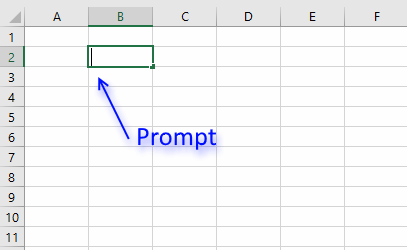An input prompt appears in the cell.
2. Begin typing an equal sign, it looks like this: =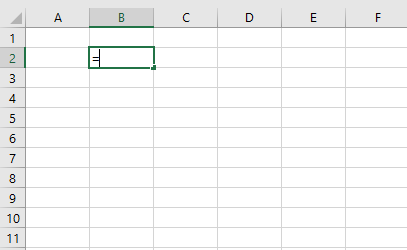This tells Excel to interpret the remaining text you type a s a formula.
3. I am going to type TODAY(), it will return the current date.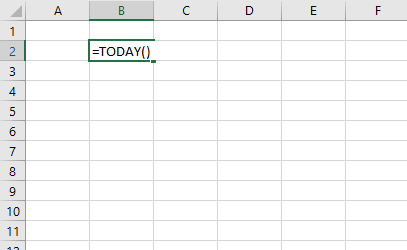Press Enter when you have finished entering the formula.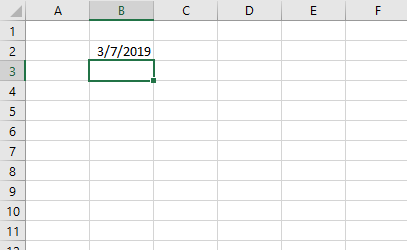### How do I create cell references in a formula?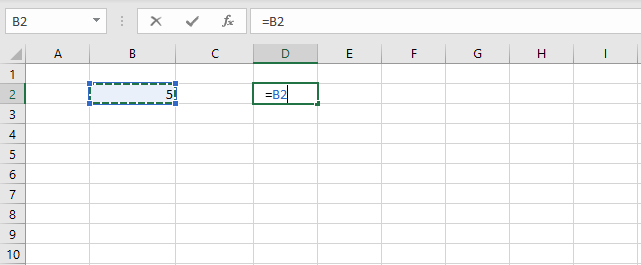You can type the cell references or you can use your mouse to click on a cell, here is how:

1. Select a cell.
2. Type the equal sign =
3. Use your mouse and click on a cell you want to reference and the cell reference will appear automatically in the formula.

### How do I type a formula and not let Excel evaluate/calculate the formula?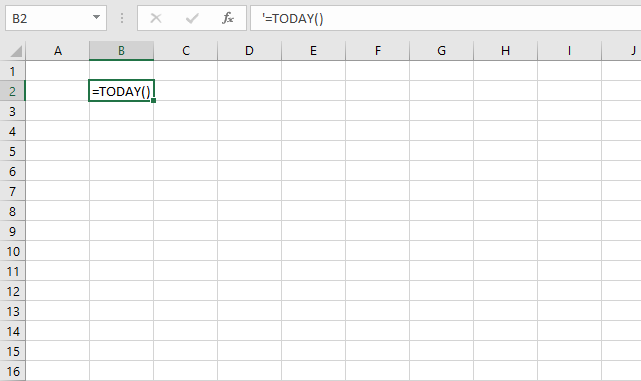Begin your formula with a ' character (apostrophe) and then type the equal sign and the formula. Excel will interpret it as a text value and the ' character (apostrophe) will not be shown in the cell. It will, however, be displayed in the formula bar.

The image above demonstrates a formula that begins with a apostrophe. You can also use the FORMULATEXT function to show the contents of a cell.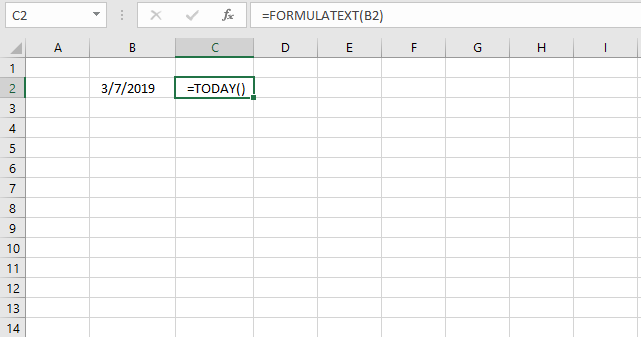### Can I combine functions in a formula?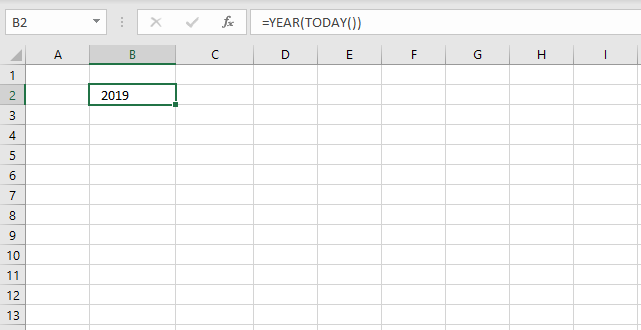Yes, and I highly recommend it. This makes Excel so powerful and interesting to use, you can customize the formulas to your needs.

The image above demonstrates two functions TODAY function and YEAR function. The TODAY function returns an Excel date that Excel formats as a date. The YEAR function then extracts the year from the Excel date.

### Can a formula return multiple values?

Yes, in fact there are a few functions that actually return multiple values by design. They return values distributed over a number of cells and they require you to enter them as an array formula in order to show all values.

You can also create an array formula that uses regular functions, however, it returns multiple values. This may seem confusing but most regular functions can be used in array formulas.

The new TEXTJOIN function can also concatenate multiple values and return them to single cell.

### How do I add two numbers?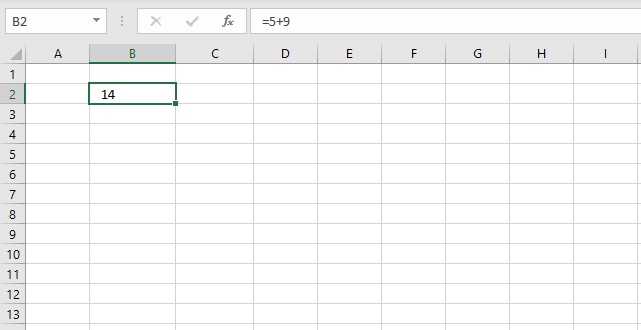Simply type =5+9 in a cell and then press Enter. Excel returns the calculated value and the Formula bar shows the arithmetic operation.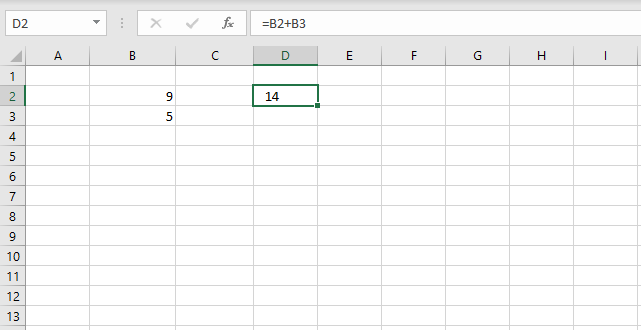You can also use cell references pointing to different cells, the image above demonstrates two numbers 9 and 5 in cell B2 and B3 respectively. The formula in cell D2 adds the numbers in cell B2 and B3 based on cell references.

### How do I divide two numbers?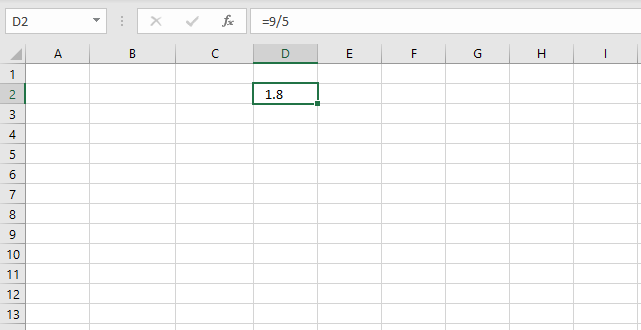Use the forward slash character / to divide numbers.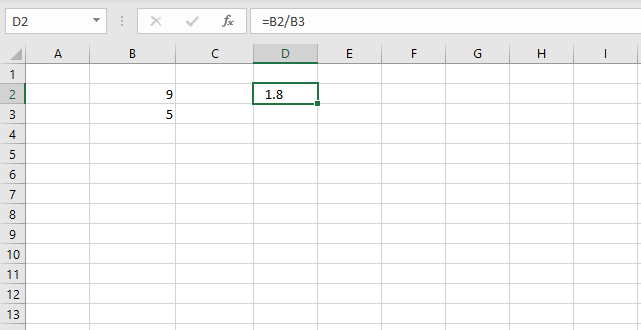Replace the numbers with cell references to cells containing the numbers you want to divide.

### How do I subtract a numbers?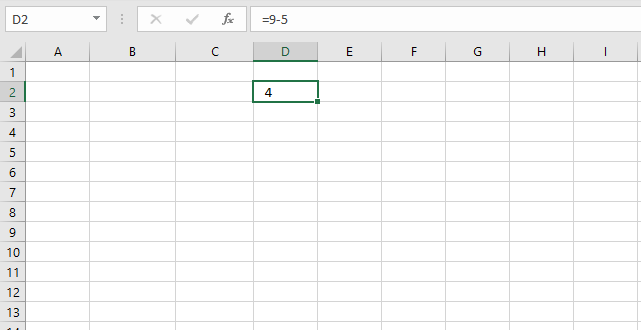Use the forward slash character / to divide numbers.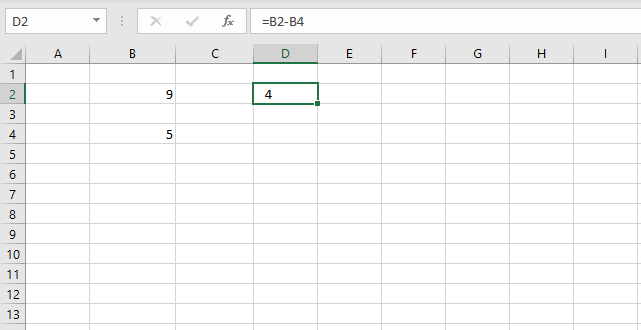Replace the numbers with cell references to cells containing the numbers you want to divide.

### How can I multiply two numbers?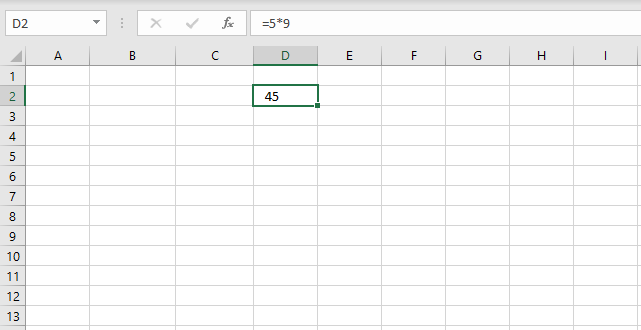Use the asterisk character * to multiply numbers.Replace the numbers with cell references to cells containing the numbers you want to multiply.

### How can I sum numbers in a cell range using a formula?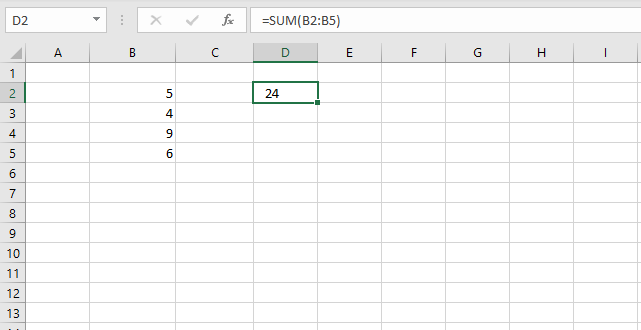1. Select the cell where you want the formula.
2. Type the equal sign =
3. Type SUM(
4. Use your mouse and click and hold on a cell containing the first number you want to add.
5. Drag with mouse to include the remaining cells.
6. Release mouse button.
7. Type an ending parentheses )
8. Press Enter.

### How can I sum numbers in a cell range based on a condition?

Use the SUMIFS function.

### How can I multiply numbers in a cell range?

Use the PRODUCT function.

### How do I calculate the power of a number (number is raised to a power) ?

Use the POWER function or simply use this character ^. Example =10^2 returns 100.

### How do I round a number up?

I recommend that you try the ROUNDUP function.

### How do I round a number down?

I recommend that you try the ROUNDDOWN function.

### What is an Excel date?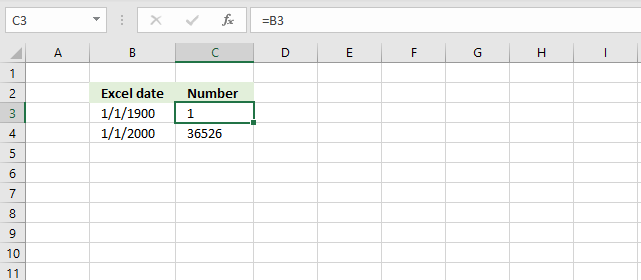It is actually an integer from 1 and up. 1 is 1/1/1900 and 1/1/2000 is 36526 so 1/1/2000 is 36525 days from 1/1/1900.

Try it yourself, enter a date in a cell. Select the cell containing the date and then press CTRL + 1 to format the cell.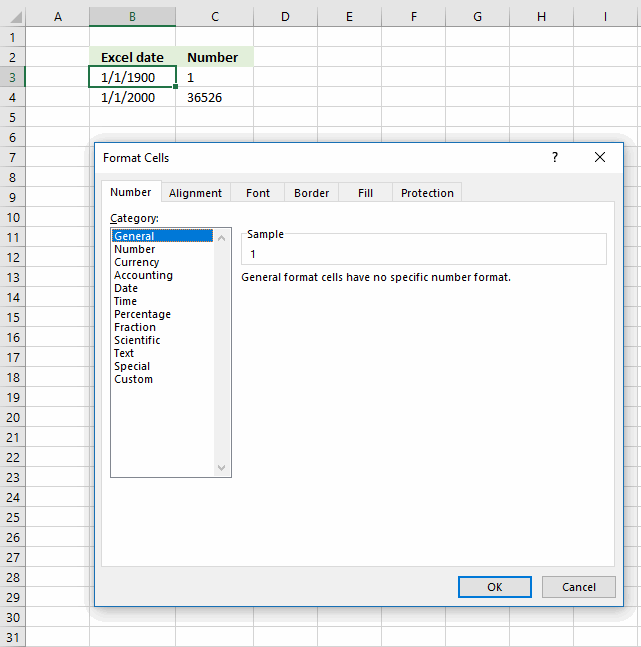Change the formatting to "General". Click OK.The cell now shows the number representing the date.

### How can I get the current date?

Use the TODAY() function.

### Can I add days to a date?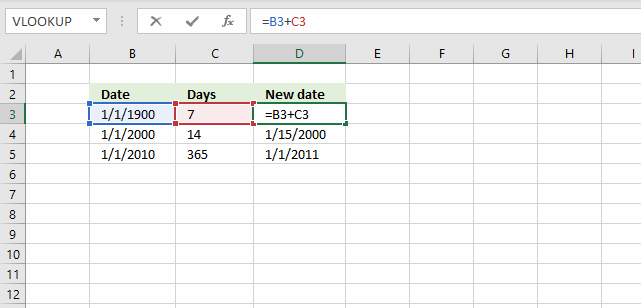Yes, since dates are integers you simply add a number representing days to the date. The image above displays 1/1/1900 in cell B3, cell C3 contains 7.

The formula in cell D3 is =B3+C3, it adds 7 to 1/1/1900 and returns 1/8/1900.

### Can I subtract days to a date?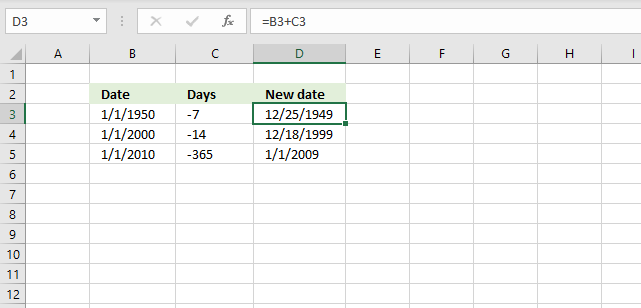The image demonstrates the same formula as the previous example above, however it uses negative numbers as dates instead.

### How can I get the current time?

The NOW function returns the current date and time, however the TEXT function can format the value to show only the time part. Use the following formula: =TEXT(NOW(),"HH:ss")

### How can I add hours to a time value?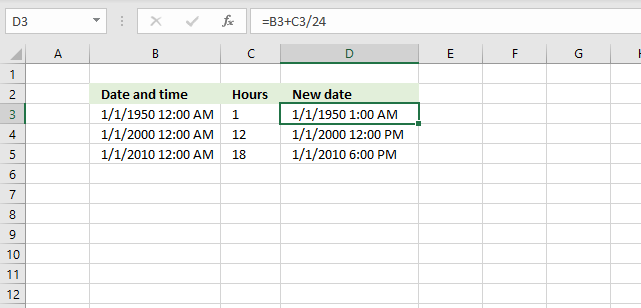1 day or 24 hours is 1 in Excel, 1 hour is 1/24 so to add 1 hour simply add 1/24 to the Excel date and time value.

### Can a formula count values?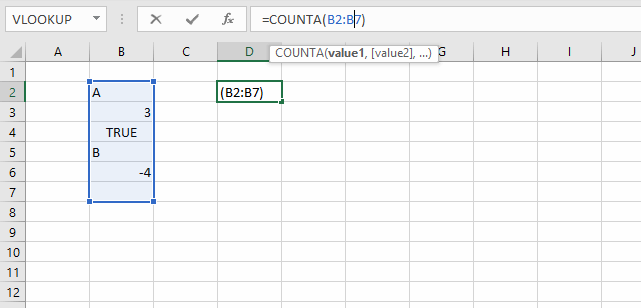Yes, the COUNTA function counts non-empty values in a given cell range.

### Can a formula count values based on a condition?

Yes, use the COUNTIF function.

### Can a formula count empty cells?

Yes, use the COUNTBLANK function.

### Can a formula count cells containing only numbers?

Yes, use the COUNT function.

### Can a formula count text values?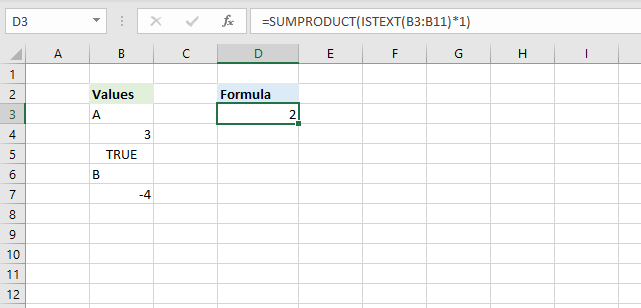Yes, the image above shows a formula in cell D3 that counts text values in cell range B3:B11.

=SUMPRODUCT(ISTEXT(B3:B11)*1)

The ISTEXT function returns TRUE if a cell contains a text value and FALSE if not. The SUMPRODUCT function simply adds the values and returns the total.

### Can a formula count boolean values?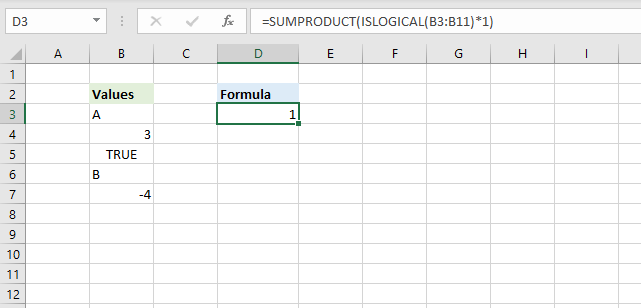Yes, the image above shows a formula in cell D3 that counts logical values in cell range B3:B11.

=SUMPRODUCT(ISLOGICAL(B3:B11)*1)

The ISLOGICAL function returns TRUE if a cell contains a boolean value and FALSE if not. The SUMPRODUCT function simply adds the values and returns the total.

### Can a I use parentheses in a formula?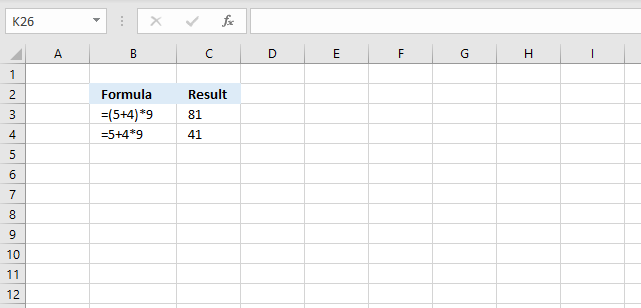Yes, use it to change the order of calculation. The picture above shows two formulas, the parentheses determines the order of calculation.

The formula in cell C3 calculates 5+4 first and then multiplies with 9. 9*9 = 81

The formula in cell C4 calculates the multiplication first and then the addition. 4*9 equals 36 and then adds 5 equals 41.

### What is a logical expression?

It is an expression that returns either TRUE or FALSE or their equivalents 1 or 0 (zero). It uses the comparison operators in order to determine the outcome.

Example formula: =B3>5

The formula above returns TRUE if the value in cell B3 is larger than 5 and FALSE if it is equal to or smaller than 5.

A logical expression is used in IF functions, Conditional Formatting and in SUMPRODUCT formulas to name a few.

### What is a comparison operator?

It is a character that compares two or more values and is used in logical expressions. They are:

• <
• >
• =

With these three characters you can create logical operators:

• < - Less than
• > - Larger than
• = - Equal to
• <= - Less than or equal to
• >= - Larger than or equal to
• <> - Not equal to

### How do I calculate sine?

Use the SIN function.

### How do I calculate cosine?

Use the COS function.

### How do I calculate tangent?

Use the TAN function.

### How do I calculate secant?

Use the SEC function.

### How do I calculate cosecant?

Use the CSC function.

### How do I calculate cotangent?

Use the COT function.

### How do I remove the sign before a number?

The ABS function allows you to delete the minus sign.

#### Latest articles in Basic formulas category

How to sum a cell range

The easiest way to sum a cell range is to simply select the cell range and read the values in […]

Sum unique distinct numbers

The image above shows numbers in column B, some of these numbers are duplicates. The formula in D12 adds unique […]

INDEX MATCH – Last value

INDEX and MATCH are more versatile than the VLOOKUP function in terms of lookups, however, it only gets the first […]

How to count blank cells

The image above shows the COUNTBLANK function counting empty cells in cell range B3:B14. Note that the COUNTBLANK function ignores […]

Count complete hours between two times

The formula in cell D5 calculates the number of complete hours between the time entries in cell B5 and C5. […]

How to calculate the number of weeks between dates

The image above demonstrates a formula that calculates the number of complete weeks between two dates. Column B and column C […]

How to calculate the number of months between dates

The image above shows the DATEDIF function calculating the number of complete months between two dates. Column B and column C […]

How to calculate the number of years between dates

The image above demonstrates the DATEDIF function calculating the number of complete years between two dates. Column B and column C […]

How to count workdays between two dates

Excel contains a great function that easily counts the number of workdays between two dates, it even allows you to […]

How to replace part of formula in all cells

This article explains how to substitute part of a formula across all cells in a worksheet. It is easier than […]

If cell has value

The easiest way to check if a cell has a value is, in my opinion, to use the equal sign […]

Count a given pattern in a cell value

The formula in cell B6 counts how many times the string (D3) is found in a cell value (B3) even […]

Extract k-th word in cell value

The formula displayed above in cell range D3:D9 extracts a word based its position in a cell value. For example, […]

If cell contains any text

The picture above shows different values in column B and a formula in column C that tries to identifies the […]

Count cells with text

The following formula in cell D3 counts cells with values stored as text. =SUMPRODUCT(ISTEXT(B3:B14)*1) In other words, cells containing nothing, errors, […]

Count rows with data

The formula in cell B17 counts rows in cell range B3:D17 when at least one cell per row contains data. […]

Count cells equal to any value in a list

The formula in cell F9 counts the number of cells in column B (Values1) that are equal to any of the […]

Get the latest revision

Column B contains document names, many of them are duplicates. The adjacent column C has the revision of the documents […]

How to extract numbers from a cell value

The following array formula, demonstrated in cell C3, extracts all numbers from a cell value: =TEXTJOIN(, 1, TEXT(MID(B3, ROW(\$A\$1:INDEX(\$A\$1:\$A\$1000, LEN(B3))), […]

How to remove unwanted characters in a cell

Cell B3 contains a few odd characters and the formula in C3 shows the ANSI equivalent of each character in […]

Count specific WEEKDAYS between two dates

If you want to count specific weekdays like for example Mondays and Wednesdays you need a more complicated array formula. […]

Extract last word in cell

The formula demonstrated above in cell range C3:C9 extracts the last word from adjacent cell in column B. =TRIM(RIGHT(SUBSTITUTE(B3, " […]

How to remove numbers from a cell value

The array formula in cell C3:C7 extracts everything except numbers from cell B3. The following formula contains the TEXTJOIN function […]

Extract first word in cell

The formula in cell C3 grabs the first word in B3 using a blank as the delimiting character. =LEFT(B3,SEARCH(" ",B3)-1) […]

LEFT function for numbers

The LEFT function allows you to extract a string from a cell with a specific number of characters, however, if […]

INDEX and MATCH – multiple criteria and multiple results

The formula in cell C14 returns multiple values from column Item. It uses multiple criteria specified in C12:C13 and applied […]

Count cells containing text from list

The array formula in cell F3 counts cells in column B that contains at least one of the values in […]

If cell contains multiple values

The array formula in cell C3 checks if text string in B3 contains all values in F2:F3.

Days between two dates

The DATEDIF function in cell E3 allows you to calculate days between two dates.

SMALL function – INDEX MATCH

The array formula in cell C11 gets 3 values in one fetch, the INDEX function allows you to do that […]

How to AVERAGE time

Column C contains both date and time, to calculate the average of only time we need to extract the hours, minutes […]

INDEX MATCH – Case sensitive

The picture above demonstrates a formula in cell F3 that allows you to look up a value in column B […]

INDEX MATCH – multiple results

The array formula in cell E6 extracts values from column C when the corresponding value in column B matches the […]

Match two columns

The array formula in cell D12 matches two values in two columns each and returns a value on the same […]

If cell equals value from list

Regular formula Formula in cell C3: =IF(COUNTIF(\$E\$3:\$E\$5,B3),"Yes","No") The COUNTIF function counts how many values in E3:E5 match cell B3, it returns […]

Sum by group

To extract groups from cell range B3:B10 I use the following regular formula in cell B13.

If cell contains text from list

This article demonstrates several ways to check if a cell contains a value based on a list. The first example […]

INDEX MATCH with multiple criteria

The formula demonstrated in cell D13 is a regular formula, most people prefer a regular formula over an array formula […]

Sum numbers between two dates

The formula in cell C15 uses two dates two to filter and then sum values in column C, the SUMIFS […]

If cell contains text

The easiest way to check if a cell contains a specific text string is, in my opinion, the IF and […]

Use IF + COUNTIF to perform numerous conditions

The COUNTIF function allows you to construct a small IF formula that carries out plenty of logical expressions. Combining the IF […]

IF with AND function – multiple conditions

The AND function allows you to have multiple conditions in an IF function, you can have up to 254 arguments. […]

Nested IF functions

Nested IF statements in a formula are multiple combined IF functions so more conditions and outcomes become possible. They all are […]

Running totals

The SUM function in cell D3 uses only a single cell reference and still manages to sum current and previous […]

Find empty cells and sum cells above

Is it possible to quickly select all empty cells and then sum cells above to next empty cell? Can I have […]

Find last value in a column

The formula in cell D3 lets you get the last value in column B, it works fine with blank cells […]

How to calculate overlapping time ranges

I found an old post that I think is interesting to write about today. Think of two overlapping ranges, it may be dates, […]

Find last matching value in an unsorted list

I read an interesting blog post Find Last Item in Group With Index Match written by Debra Dalgleish. It is about […]

Lookup with an unknown number of criteria

Rashid asks:I used your array formula with great success to find the search results from multiple criteria. However, my problem […]

Count multiple text strings in a cell range

This article demonstrates an array formula that counts how many times multiple text strings exist in a cell range.  The […]

How to create name initials

The array formula in cell C3 extracts the first character from first, middle and last name. The formula works fine […]

Extract table headers based on a condition

This article demonstrates an array formula that returns the table header based on a condition. For example, in cell C8 […]

Lookup multiple values across columns and return a single value

This article demonstrates how to get a value from a dataset based on multiple conditions across multiple columns. S.Babu asks: […]

Find entry based on conditions

Bill Truax asks: Hello Oscar, I am building a spreadsheet for tracking calls for my local fire department. I have a […]

Extract numbers from a value

Chirag asks: I want to separate numbers from the following text: Abc123bx45 as a result 123 and 45 should be […]

Sum cells based on criteria

Katie asks: I have 57 sheets many of which are linked together by formulas, I need to get numbers from […]

7 days (weekly) date ranges using a formula

Shannon asks:I need a formula that if I enter a start date in field B1 such as 6/8/11 it will […]

Running totals based on criteria

Andrew asks: LOVE this example, my issue/need is, I need to add the results. So instead of States and Names, […]

Sum based on OR – AND logic

Question: It's easy to sum a list by multiple criteria, you just use array formula a la: =SUM((column_plane=TRUE)*(column_countries="USA")*(column_producer="Boeing")*(column_to_sum)) But all […]

Formula for matching a date within a date range

Table of contents Match a date when a date range is entered in a single cell Match a date when […]

Find latest date based on a condition

Sum values between two dates and based on a condition

In this post, I will provide a formula to sum values in column (Qty) where a column (Date) meets two […]

Lookup two index columns

Formula in B14: =INDEX(D3:D6, SUMPRODUCT(--(C10=B3:B6), --(C11=C3:C6), ROW(D3:D6)-MIN(ROW(D3:D6))+1)) Alternative array formula #1 in B15: =INDEX(D3:D6, MATCH(C10&"-"&C11, B3:B6&"-"&C3:C6, 0)) Alternative array formula […]

Extract numbers from a column

I this article I will show you how to get numerical values from a cell range manually and using an […]

List values with past date

Question: I have a column "B" with a last name.. I have another columb with a date in it "C"... […]

Count text string in a range (case sensitive)

Question: How do I count the number of times a text string exists in a column? The text string may […]

Count specific text string in a cell

Question: How do I count how many times a text string exists in a cell value in Excel? Answer: The […]

How to perform a two-dimensional lookup

Question: How would I go about looking up data in a cross-reference table. I have the header row (i.e. 24) […]

Create a date range [Formula]

Question: I am trying to create an excel spreadsheet that has a date range. Example: Cell A1 1/4/2009-1/10/2009 Cell B1 […]

Sum unique numbers

The formula in cell D3 adds all unique numbers in cell range B3:B12 and returns the total. Unique values are all […]

Count dates inside a date range

How do I automatically count dates in a specific date range? Array formula in cell D3: =SUM(IF((\$A\$2:\$A\$10<\$D\$2)*(\$A\$2:\$A\$10>\$D\$1), 1, 0)) + […]

Count how many times a string exists in a cell range (case insensitive)

Question: How do I count how many times a word exists in a range of cells? It does not have […]

Find closest value